#### 期刊菜单

Analysis of Temperature Field of Three-Core Submarine Cable Based on Finite Element Simulation
DOI: 10.12677/JEE.2022.101004, PDF, HTML, XML, 下载: 119  浏览: 241

Abstract: With the development of offshore wind power, submarine oil and natural gas, submarine cables play an important role in power transmission, but a large amount of heat is generated during operation, which has many adverse effects on power transmission. Therefore, the analysis of the temperature field of submarine cables is worthy of attention. At present, scholars at home and abroad have also conducted a lot of research on the current carrying capacity and temperature field of submarine cables. In this paper, the ANSYS finite element simulation software is used to calculate the temperature field of the three-core optical fiber composite submarine cable, and simulate the soil in different seasons and different sea areas. In this paper, the influence of different ambient temperatures, different soil thermal conductivity and different burial depths on the temperature field of the three-core submarine cable during direct burial is analyzed. The results show that the conductor temperature is positively correlated with the ambient temperature, and the conductor temperature increases by about 3°C for every 4°C increase in ambient temperature; positive correlation with burial depth; negative correlation with soil thermal conductivity. It provides a reference for the design of the three-core submarine cable and the planning of the submarine cable transmission line.

1. 引言

2. 基于ANSYS的海缆仿真模型

2.1. 海缆的物理模型与简化

35 kV高压三芯交联聚乙烯光纤复合海缆的截面结构如图1所示，主要由三个线芯、两个光纤单元及外层材料组成，其中，光纤单元作为分布式光纤测温的媒介，对于海缆温度监测起着重要作用，从内到外依次为光纤、不锈钢管、钢丝加强、绕包包带与PE护套；线芯从内到外依次为阻水铜导体、导体屏蔽、XLPE绝缘层、绝缘屏蔽、半导电阻水带、合金护套、半导电PE护套；外层材料从内到外依次为填充层、包带、PP内垫层、镀锌钢丝铠装层、沥青及PP外被层。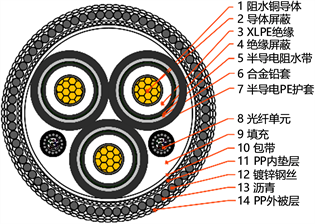Figure 1. Cross-sectional view of fiber optic composite submarine cable structureFigure 2. Simplified submarine cable modelTable 1. Size and structure parameters of each layer of submarine cable

1) 电缆各层的内外表面为等温面；

2) 电缆的几何参数为常数；

3) 仅考虑导体损耗、绝缘层损耗、合金铅套损装耗和铠装损耗；

4) 不计导体屏蔽层﹑绝缘屏蔽层、铠装层热阻。

2.2. 海缆温度场有限元分析模型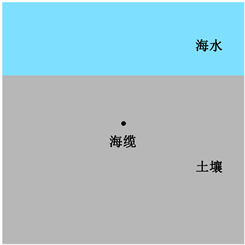Figure 3. Submarine cable direct buried laying diagram

2.3. 有限元模型边界条件设定

$\rho c\frac{\partial T}{\partial \tau }=\frac{\partial }{\partial x}\left(\kappa \frac{\partial T}{\partial x}\right)+\frac{\partial }{\partial x}\left(\kappa \frac{\partial T}{\partial y}\right)+{q}_{v}$ (1-1)

$\left\{\begin{array}{l}{T|}_{\Gamma }={T}_{w}\\ {T|}_{\Gamma }=f\left(x,y,t\right)\end{array}$ (1-2)

$\left\{\begin{array}{l}{\kappa \frac{\partial T}{\partial n}|}_{\Gamma }+{q}_{\theta }=0\\ {\kappa \frac{\partial T}{\partial n}|}_{\Gamma }+g\left(x,y,t\right)=0\end{array}$ (1-3)

$\left\{-{\kappa \frac{\partial T}{\partial n}|}_{\Gamma }={\alpha \left(T-{T}_{f}\right)|}_{\Gamma }$ (1-4)

2.4. 有限元模型网格划分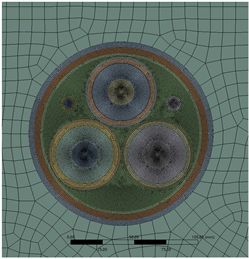Figure 4. Submarine cable meshing

3. 海缆温度场仿真结果与分析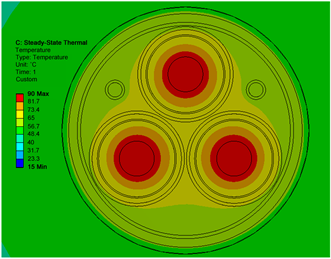(a)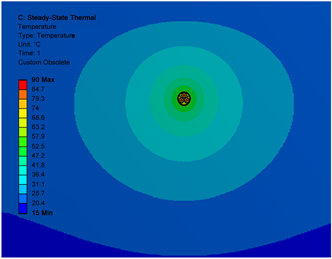(b)

Figure 5. Cloud map of temperature distribution (a) Local temperature distribution of submarine cable; (b) Global temperature distributionTable 2. Corresponding relationship between ambient temperature and conductor temperature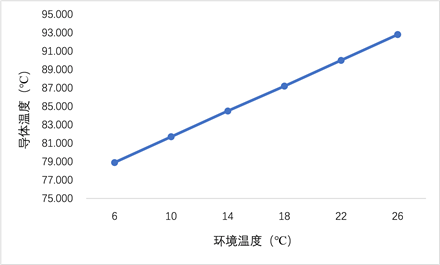Figure 6. Corresponding relationship between ambient temperature and submarine cable conductor temperatureTable 3. Corresponding relationship between soil thermal conductivity and conductor temperature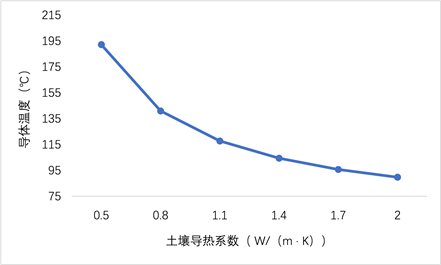Figure 7. Corresponding relationship between soil thermal conductivity and submarine cable conductor temperatureTable 4. Corresponding relationship between submarine cable burial depth and submarine cable conductor temperature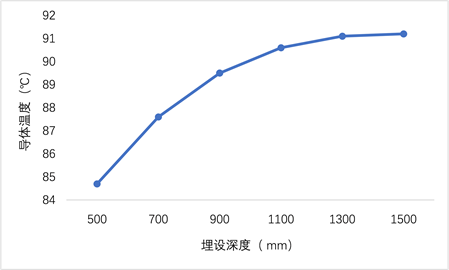Figure 8. Corresponding relationship between submarine cable burial depth and submarine cable conductor temperature

4. 结论

  李萌, 牛胜锁, 刘玉芹, 赵可为. 基于多场耦合模型的海底电缆载流量和温度场计算研究[J]. 电测与仪表, 2018, 55(8): 12-16+23.  张巍伟. 基于解析法和有限元法的海底电缆温度场分析[J]. 船电技术, 2020, 40(10): 40-44.  刘贺晨, 张铭嘉, 刘云鹏, 刘爱静, 徐晓彬. ±160 kV交联聚乙烯直流海底电缆动态载流特性试验及仿真研究[J]. 电测与仪表, 2020, 57(14): 19-27.  张磊, 宣耀伟, 乐彦杰, 张占奎, 李琰, 沈耀军. 110 kV单芯海缆的载流量计算、温度场仿真及其热循环试验研究[J]. 高压电器, 2016, 52(6): 135-140+146.  刘英, 张申冬, 曹俊平, 王少华. 500 kV直流XLPE海缆的热场及电场仿真研究[J]. 高压电器, 2020, 56(12): 7-15.  段佳冰, 尹成群, 吕安强, 李永倩. 基于IEC 60287和有限元法的高压海底电缆温度场分析方法[J]. 高压电器, 2014, 50(1): 1-6.  刘云鹏, 许自强, 陈铮铮, 刘贺晨. ±160 kV直流XLPE海底电缆载流特性仿真及试验[J]. 电力自动化设备, 2018, 38(2): 130-136.  郝艳捧, 陈云, 阳林, 黄吉超, 傅明利, 王邸博, 侯帅. 高压直流海底电缆三维仿真模型简化等效研究[J]. 南方电网技术, 2017, 11(4): 1-6.  Zhou, B.D., Le, Y.J., Fang, Y.L., Yang, F., Dai, T., Sun, L., Liu, J.P. and Zou, L.K. (2018) Temperature Field Simulation and Ampacity Optimisation of 500 kV HVDC Cable. The Journal of Engineering, 2019, 2448-2453. https://doi.org/10.1049/joe.2018.8586  杨其鹏. 考虑不同接头影响下的海底电缆温度场分析与载流量计算[D]: [硕士学位论文]. 重庆: 重庆大学, 2017.  Gela, G. and Dai, J. (1988) Calculation of Thermal Fields of Underground Cables Using the Boundary Element Method. IEEE Transactions on Power Delivery, 5, 1341-1347. https://doi.org/10.1109/61.193929  Hiranandani, A. (1991) Calculation of Conductor Temperatures and Ampacities of Cable Systems Using a Generalized Finite Difference Model. IEEE Transactions on Power Delivery, 6, 15-21. https://doi.org/10.1109/61.103717  秦春旭, 栗丙典, 李国明, 杨晓辉, 林长海. 基于有限元对220 kV三芯海缆温度场的数值计算[J]. 东北电力大学学报, 2019, 39(4): 5-10.  吴浩. 基于计算机技术的海缆温度场建模及应用研究[J]. 粘接, 2021, 48(10): 76-80.